Chapter 15. Nuclear Chemistry

Learning Objectives

1. Define and give examples of the major types of radioactivity.

We saw in Chapter 3 “Atoms, Molecules, and Ions” that atoms are composed of subatomic particles — protons, neutrons, and electrons. Protons and neutrons are located in the nucleus and provide most of the mass of an atom, while electrons circle the nucleus in shells and subshells and account for an atom’s size.

We also introduced in Chapter 3 the notation for succinctly representing an isotope of a particular atom: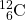The element in this example, represented by the symbol C, is carbon. Its atomic number, 6, is the subscript next to the symbol and is the number of protons in the atom. The mass number, the superscript next to the symbol, is the sum of the number of protons and neutrons in the nucleus of this particular isotope. In this case, the mass number is 12, which means that the number of neutrons in the atom is 12 − 6 = 6 (that is, the mass number of the atom minus the number of protons in the nucleus equals the number of neurons). Occasionally, the atomic number is omitted in this notation because the symbol of the element itself conveys its characteristic atomic number. The two isotopes of hydrogen — 2H and 3H — are given their own names and symbols: deuterium (D) and tritium (T), respectively.

Atomic theory in the nineteenth century presumed that nuclei had fixed compositions. But in 1896, the French scientist Henri Becquerel found that a uranium compound placed near a photographic plate made an image on the plate, even if the compound was wrapped in black cloth. He reasoned that the uranium compound was emitting some kind of radiation that passed through the cloth to expose the photographic plate. Further investigations showed that the radiation was a combination of particles and electromagnetic rays, with its ultimate source being the atomic nucleus. These emanations were ultimately called, collectively, radioactivity.

There are three main forms of radioactive emissions. The first is called an alpha particle, which is symbolized by the Greek letter α. An alpha particle is composed of two protons and two neutrons and is the same as a helium nucleus. (We often use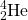to represent an alpha particle.) It has a 2+ charge. When a radioactive atom emits an alpha particle, the original atom’s atomic number decreases by two (because of the loss of two protons), and its mass number decreases by four (because of the loss of four nuclear particles). We can represent the emission of an alpha particle with a chemical equation—for example, the alpha-particle emission of uranium-235 is as follows: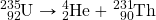Rather than calling this equation a chemical equation, we call it a nuclear equation to emphasize that the change occurs in an atomic nucleus. How do we know that a product of this reaction is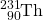? We use the law of conservation of matter, which says that matter cannot be created or destroyed. This means we must have the same number of protons and neutrons on both sides of the nuclear equation. If our uranium nucleus loses 2 protons, there are 90 protons remaining, identifying the element as thorium. Moreover, if we lose four nuclear particles of the original 235, there are 231 remaining. Thus we use subtraction to identify the isotope of the Th atom — in this case,.

Chemists often use the names parent isotope and daughter isotope to represent the original atom and the product other than the alpha particle. In the previous example,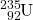is the parent isotope, andis the daughter isotope. When one element changes into another in this manner, it undergoes radioactive decay.

Example 15.5

Write the nuclear equation that represents the radioactive decay of radon-222 by alpha particle emission and identify the daughter isotope.

Solution
Radon has an atomic number of 86, so the parent isotope is represented as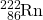. We represent the alpha particle asand use subtraction (222 − 4 = 218 and 86 − 2 = 84) to identify the daughter isotope as polonium: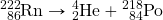Test Yourself
Write the nuclear equation that represents radioactive decay of polonium-208 by alpha particle emission and identify the daughter isotope.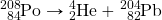; daughter isotope: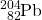The second major type of radioactive emission is called a beta particle, symbolized by the Greek letter β. A beta particle is an electron ejected from the nucleus (not from the shells of electrons about the nucleus) and has a 1− charge. We can also represent a beta particle as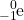. The net effect of beta particle emission on a nucleus is that a neutron is converted to a proton. The overall mass number stays the same, but because the number of protons increases by one, the atomic number goes up by one. Carbon-14 decays by emitting a beta particle: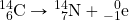Again, the sum of the atomic numbers is the same on both sides of the equation, as is the sum of the mass numbers. (Note that the electron is assigned an “atomic number” of –1, equal to its charge.)

The third major type of radioactive emission is not a particle but rather a very energetic form of electromagnetic radiation called gamma rays, symbolized by the Greek letter γ. Gamma rays themselves do not carry an overall electrical charge, but they may knock electrons out of atoms in a sample of matter and make it electrically charged (for which gamma rays are termed ionizing radiation). For example, in the radioactive decay of radon-222, both alpha and gamma radiation are emitted, with the latter having an energy of 8.2 × 10−14 J per nucleus decayed: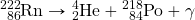This may not seem like much energy, but if 1 mol of Rn atoms were to decay, the gamma ray energy would be 4.9 × 107 kJ!

Example 15.6

Write the nuclear equation that represents the radioactive decay of boron-12 by beta particle emission and identify the daughter isotope. A gamma ray is emitted simultaneously with the beta particle.

Solution
The parent isotope is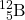, while one of the products is. So that the mass and atomic numbers have the same value on both sides, the mass number of the daughter isotope must be 12, and its atomic number must be 6. The element having an atomic number of 6 is carbon. Thus the complete nuclear equation is as follows: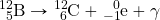The daughter isotope is carbon-12.

Test Yourself
Write the nuclear equation that represents the radioactive decay of technetium-133 by beta particle emission and identify the daughter isotope. A gamma ray is emitted simultaneously with the beta particle.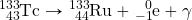; daughter isotope: ruthenium-133

Alpha, beta, and gamma emissions have different abilities to penetrate matter. The relatively large alpha particle is easily stopped by matter (although it may impart a significant amount of energy to the matter it contacts). Beta particles penetrate slightly into matter, perhaps a few centimetres at most. Gamma rays can penetrate deeply into matter and can impart a large amount of energy into the surrounding matter. Table 15.5 “The Three Main Forms of Radioactive Emissions” summarizes the properties of the three main types of radioactive emissions.

Table 15.5 The Three Main Forms of Radioactive Emissions
Characteristic Alpha Particles Beta Particles Gamma Rays
symbols α,β,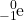γ
identity helium nucleus electron electromagnetic radiation
charge 2+ 1− none
mass number 4 0 0
penetrating power minimal (will not penetrate skin) short (will penetrate skin and some tissues slightly) deep (will penetrate tissues deeply)

Occasionally, an atomic nucleus breaks apart into smaller pieces in a radioactive process called spontaneous fission (or fission). Typically, the daughter isotopes produced by fission are a varied mix of products, rather than a specific isotope as with alpha and beta particle emission. Often, fission produces excess neutrons that will sometimes be captured by other nuclei, possibly inducing additional radioactive events. Uranium-235 undergoes spontaneous fission to a small extent. One typical reaction is: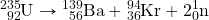where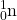is a neutron. As with any nuclear process, the sums of the atomic numbers and mass numbers must be the same on both sides of the equation. Spontaneous fission is found only in large nuclei. The smallest nucleus that exhibits spontaneous fission is lead-208. (Fission is the radioactive process used in nuclear power plants and one type of nuclear bomb.)

Key Takeaways

• The major types of radioactivity include alpha particles, beta particles, and gamma rays.
• Fission is a type of radioactivity in which large nuclei spontaneously break apart into smaller nuclei.

Exercises

# Questions

2. Give an example of a radioactive element. How do you know if it is radioactive?
3. How many protons and neutrons are in each isotope?
1.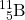2.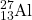3.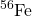4.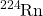4. How many protons and neutrons are in each isotope?
1.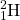2.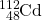3.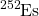4.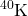5. Describe an alpha particle. What nucleus is it equivalent to?
6. Describe a beta particle. What subatomic particle is it equivalent to?
7. What are gamma rays?
8. Why is it inappropriate to refer to gamma rays as “gamma particles”?
9. Plutonium has an atomic number of 94. Write the nuclear equation for the alpha particle emission of plutonium-244. What is the daughter isotope?
10. Francium has an atomic number of 87. Write the nuclear equation for the alpha particle emission of francium-212. What is the daughter isotope?
11. Tin has an atomic number of 50. Write the nuclear equation for the beta particle emission of tin-121. What is the daughter isotope?
12. Technetium has an atomic number of 43. Write the nuclear equation for the beta particle emission of technetium-99. What is the daughter isotope?
13. Energies of gamma rays are typically expressed in units of megaelectron volts (MeV), where 1 MeV = 1.602 × 10−13 J. Using the data provided in the text, calculate the energy in megaelectron volts of the gamma ray emitted when radon-222 decays.
14. The gamma ray emitted when oxygen-19 gives off a beta particle is 0.197 MeV. What is its energy in joules? (See Exercise 13 for the definition of a megaelectron volt.)
15. Which penetrates matter more deeply — alpha particles or beta particles? Suggest ways to protect yourself against both particles.
16. Which penetrates matter more deeply — alpha particles or gamma rays? Suggest ways to protect yourself against both emissions.
17. Define nuclear fission.
18. What general characteristic is typically necessary for a nucleus to undergo spontaneous fission?

1. Radioactivity is the spontaneous emission of particles and electromagnetic radiation from nuclei of unstable atoms.
1. 5 protons; 6 neutrons
2. 13 protons; 14 neutrons
3. 26 protons; 30 neutrons
4. 86 protons; 138 neutrons
1. An alpha particle is a collection of two protons and two neutrons and is equivalent to a helium nucleus.
1. Gamma rays are high-energy electromagnetic radiation given off in radioactive decay.
1.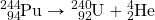; daughter isotope: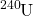1.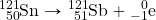; daughter isotope: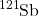1. 0.51 MeV
1. Beta particles penetrate more. A thick wall of inert matter is sufficient to block both particles.
1. Nuclear fission is the breaking down of large nuclei into smaller nuclei, usually with the release of excess neutrons.